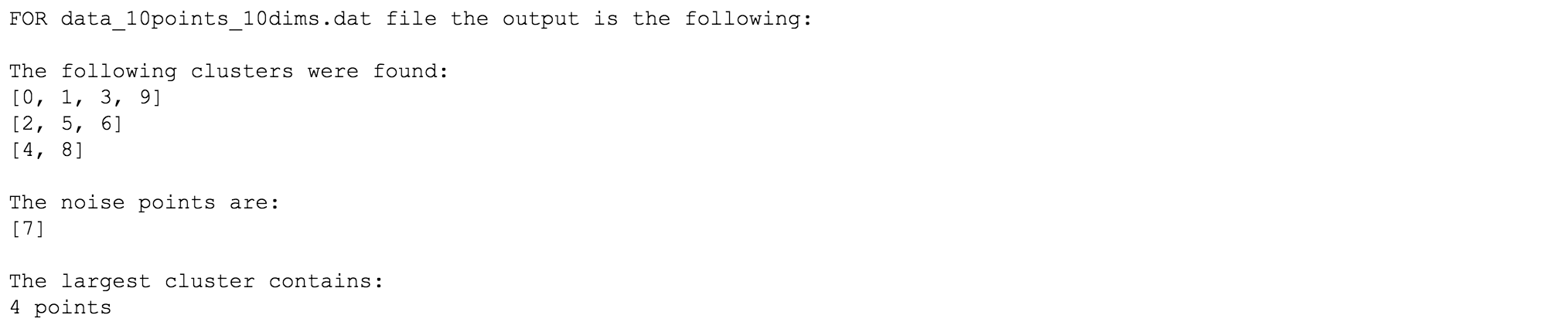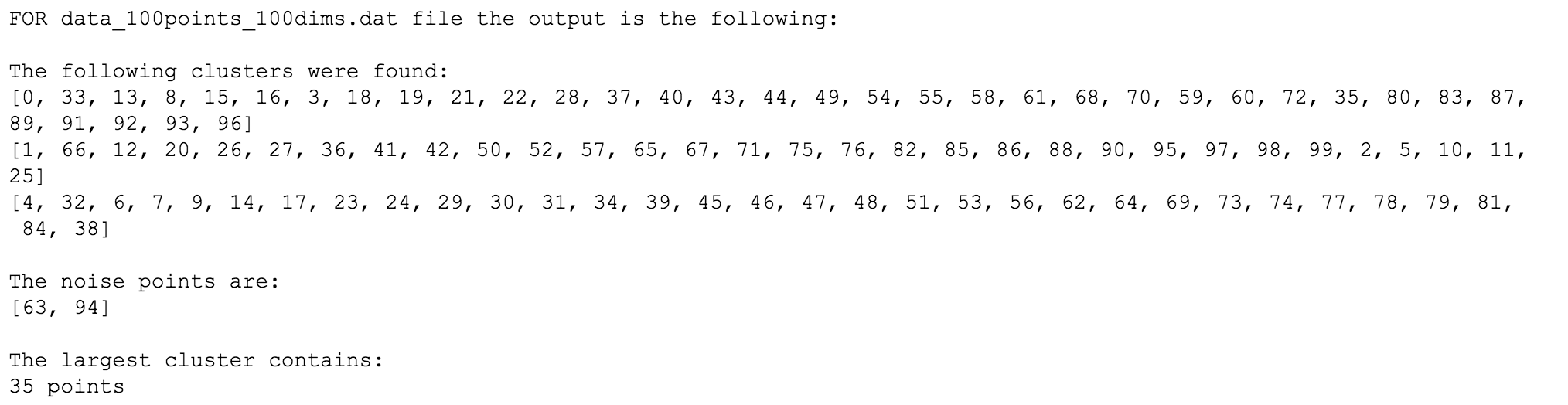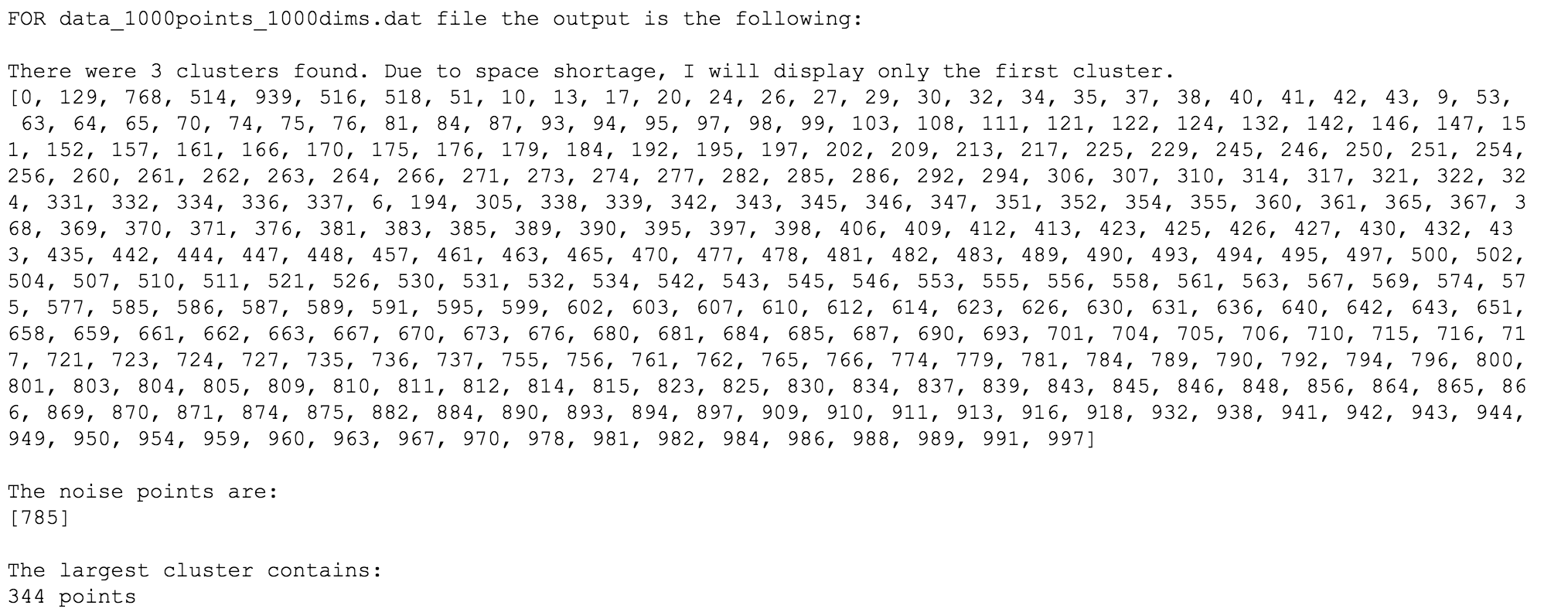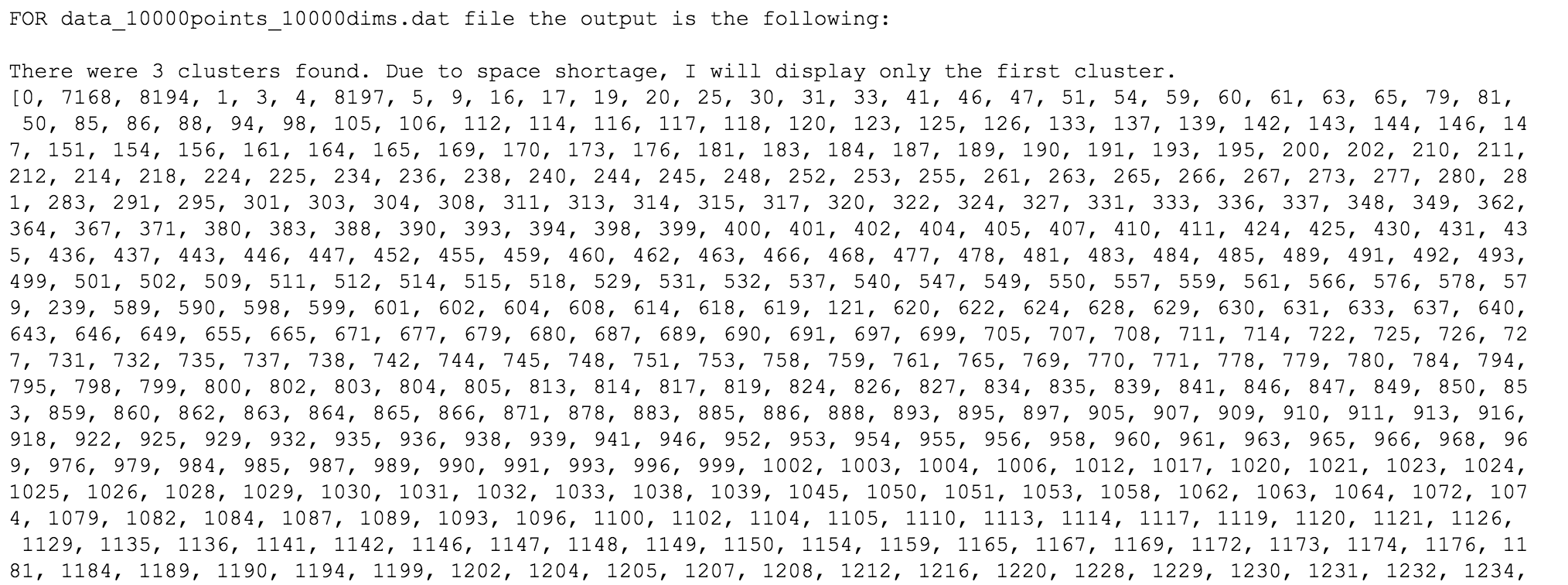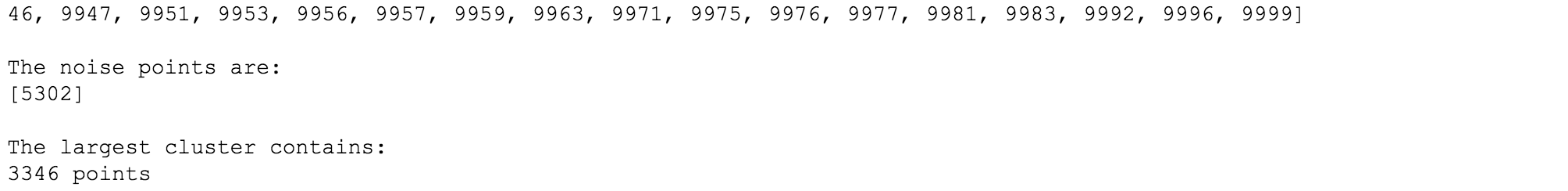In this post I describe how to implement the DBSCAN clustering algorithm to work with Jaccard-distance as its metric. It should be able to handle sparse data.

## Overview

The DBSCAN clustering algorithm will be implemented in Python as described in this Wikipedia article. The algorithm will use Jaccard-distance (1 minus Jaccard index) when measuring distance between points. In this implementation, two points that are exactly a distance epsilon apart are taken to be in the same cluster, thus greater-than-or-equal $% $ and not $% $ should be used.

This method could support a large number of sparse points in very high-dimensional space (~10,000 dimensions). This means that the points – even though they lie in very high dimensional space – only have few non-zero coordinates.

In order to test the algorithm I will use this data folder including 4 files of increasing dimensions.

• data_10points_10dims.dat
• data_100points_100dims.dat
• data_1000points_1000dims.dat
• data_10000points_10000dims.dat

Let’s have a look at the first data file, just to have in mind how it looks like.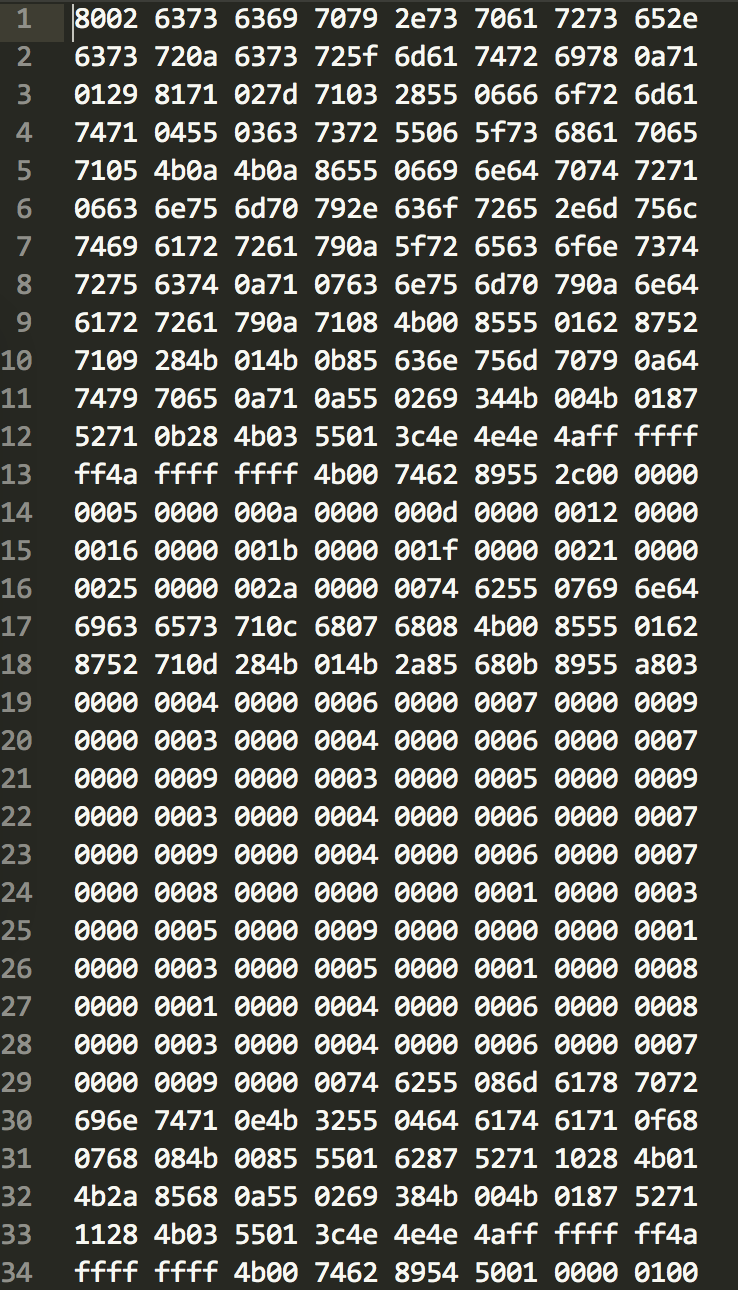Now we are ready to run the python class.

import cPickle as pickle
import numpy as np

# Create simple class used as Enum
class Status:
def __init__(self):
pass

New, Visited, Noise = range(3)

# Create variable to track the status of each point
_status = None

# Create variable to track membership of each point
_is_member = None

# Create variable to store distance between points
_distance = None

# Create variable to store indexes of ordered neighbors based on distance
_distance_idx = None;

# Create the DBSCAN algorithm as dicribed in Wikipedia
def dbscan(points, eps=0.15, min_pts=2):

clusters = []
n_pts = points.shape

# Compute the distances of the points to be clustered
_pre_compute_distance(points)

for k in range(n_pts):
if _status[k] == Status.Visited:
continue
_status[k] = Status.Visited
neighbors = _region_query(k, eps)
if len(neighbors) < min_pts:
_status[k] = Status.Noise
else:
cluster = _expand_cluster(k, neighbors, eps, min_pts)
clusters.append(cluster)

return clusters

def _expand_cluster(point, neighbors, eps, min_pts):
global _status
global _is_member

cluster = []

while neighbors:
k = neighbors.pop()
if _status[k] != Status.Visited:
_status[k] = Status.Visited
extended_neighbors = _region_query(k, eps)
if len(extended_neighbors) >= min_pts:
neighbors.update(extended_neighbors)
if not _is_member[k]:

return cluster

def _region_query(center, eps):
global _distance
# Create set instead of a list because in this case it would be necessary to iterate throught.
neighbors = set()
i = 0
# Use presorted/precomputed distances. When the closest accepted neighbors will be
# found, iteration will stops
while _distance[center, _distance_idx[center, i]] <= eps:
i += 1
return neighbors

def _pre_compute_distance(points):
global _is_member
global _status
global _distance
global _distance_idx

n_pts = points.shape

_distance = np.zeros((n_pts, n_pts))
_is_member = [False] * n_pts
_status = [Status.New] * n_pts

# Give the number of non-zero elements
n = points.sum(axis=1).dot(np.ones((1, n_pts))).A

# Compute the distance (the full array can be constructed using A + A.T)
n_intersect = points.dot(points.transpose())
_distance = 1.0 - (n_intersect / (n + n.T - n_intersect))
# The neighbors/column-indexes are sorted based on distance, to decrease the running-time
# of _range_query method
_distance_idx = np.argsort(_distance, axis=1)

cluster.append(k)
_is_member[k] = True

if __name__ == "__main__":

file_descriptor = open("test_files/data_10points_10dims.dat", "r")
_pre_compute_distance(points_to_cluster)
clusters = dbscan(points_to_cluster, eps=0.4, min_pts=2)
if len(clusters) > 0:
print "FOR data_10points_10dims.dat file the output is the following: \n"
print "The following clusters were found:"
cluster_lengths = []
for cluster in clusters:
print cluster
cluster_lengths.append(len(cluster))
noise = [point for point, status in enumerate(_status) if status == Status.Noise]
print "\nThe noise points are:\n", noise
print "\nThe largest cluster contains: \n%d points" % max(cluster_lengths)
else:
print "No clusters were found!"